# Designing Finite Automata from Regular Expression (Set 6)

In the below article, we shall see some Designing of Finite Automata form the given Regular Expression-

Regular Expression 1: Regular language,

`L1 = a(a+b)* `

The language of the given RE is,

`{aaa, aba, baa, bba} `

Its finite automata will be like below-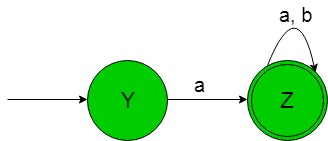In the above transition diagram, as we can see that initial state ‘Y’ on getting ‘a’ as the input it transits to a
final state ‘Z’ and so on for the remaining states. Thus this FA accepting all the strings of the given RE language.

Regular Expression 2: Regular language,

`L2 = (a+b)*a `

The language of the given RE is,

`{aaa, aba, baa, bba} `

Strings always end with ‘a’.
Its finite automata will be like below-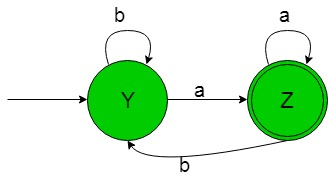In the above transition diagram, as we can see that initial state ‘Y’ on getting ‘a’ as the input it transits to a final state ‘Z’ and on getting ‘b’ as the input it remains in the state of itself and so on for the remaining states. Thus this FA accepting all the strings of the given RE language.

Regular Expression 3: Regular language,

`L3 = (a+b)*a(a+b)* `

The language of the given RE is,

`{aaa, aba, baa, bba} `

Strings containing ‘a’ as the alphabet.
Its finite automata will be like below-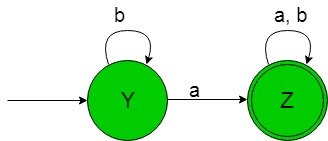In the above transition diagram, as we can see that initial state ‘Y’ on getting ‘b’ as the input it remains in the state of itself and on getting ‘a’ as the input it transits to a final state ‘Z’ and so on for the remaining states. Thus this FA accepting all the strings of the given RE language.

Regular Expression 4: Regular language,

`L4 = (a(a+b)*b)+(b(a+b)*a) `

The language of the given RE is,

`{aab, abb, baa, bba} `

Strings start and end with different symbols.
Its finite automata will be like below-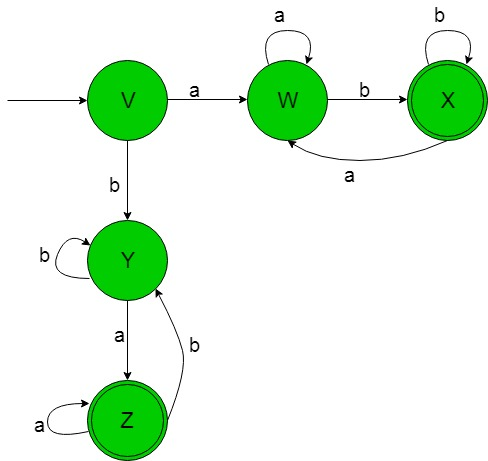In the above transition diagram, as we can see that initial state ‘V’ on getting ‘a’ as the input it transits to a state ‘W’ and so on for the remaining states. Thus this FA accepting all the strings of the given RE language.

Regular Expression 5: Regular language,

`L5 = (a(a+b)*a)+(b(a+b)*b)+a+b+ε `

The language of the given RE is,

`{&epsilon, a, b, aba, bab, bbab} `

Strings start and end with same symbols.
Its finite automata will be like below-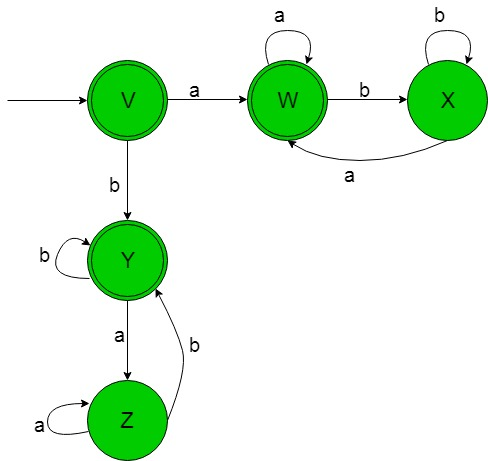In the above transition diagram, as we can see that initial and final state ‘V’ on getting ‘a’ as the input it transits to another final state ‘W’ and so on for the remaining states. Thus this FA accepting all the strings of the given RE language.

Attention reader! Don’t stop learning now. Get hold of all the important CS Theory concepts for SDE interviews with the CS Theory Course at a student-friendly price and become industry ready.

My Personal Notes arrow_drop_upCheck out this Author's contributed articles.

If you like GeeksforGeeks and would like to contribute, you can also write an article using contribute.geeksforgeeks.org or mail your article to contribute@geeksforgeeks.org. See your article appearing on the GeeksforGeeks main page and help other Geeks.

Please Improve this article if you find anything incorrect by clicking on the "Improve Article" button below.

Article Tags :

Be the First to upvote.

Please write to us at contribute@geeksforgeeks.org to report any issue with the above content.